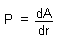HOME MATH DICTIONARY DOWNLOAD FEEDBACK DISCLAIMER
 Question: What is Perimeter ? Answer: The Perimeter of a shape is the name given to the boundary of the shape. It is also sometimes used to mean the length of the boundary. The perimeter of the building plot shown can be interpreted as its five sides or the total distance you would walk to go all the way around it: (25 + 25 + 35 + 40 + 30)m = 155m. Calculations of perimeter and area have considerable practical applications. Perimeter is used in calculating the border of an object such as a yard or flowerbed when a fence or other border is being installed around the edges. Area is used when all the area inside of a perimeter is being covered with something, such as a yard being covered with sod or fertilizer. Here is one formula how to calculate perimeter the following holds true :P stands for the perimeter d stands for the differential operator r stands for the radius A stands for the area